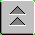Introduction to Naval Weapons Engineering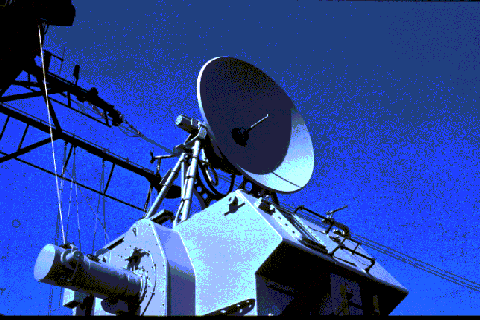Principle of Operation

refers to the use of electromagnetic waves with wavelengths in the so-called radio
wave portion of the spectrum, which covers a wide range from 104 km to 1 cm.  Radar
systems typically use wavelengths on the order of 10 cm, corresponding to frequencies
of about 3 GHz.  The detection and ranging part of the acronym is accomplished by
timing the delay between transmission of a pulse of radio energy and its subsequent
return.  If the time delay is Dt, then the range may be determined by the simple
formula:

R = cDt/2

where c = 3 x 108 m/s, the speed of light at which all electromagnetic waves propagate.
The factor of two in the formula comes from the observation that the radar pulse must
travel to the target and back before detection, or twice the range.

A radar pulse train is a type of amplitude modulation of the radar frequency
carrier wave, similar to how carrier waves are modulated in communication systems.
In this case, the information signal is quite simple:  a single pulse repeated at
regular intervals.  The common radar carrier modulation, known as the pulse train
is shown below.  The common parameters of radar as defined by referring to Figure 1.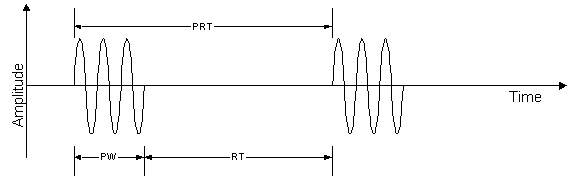PW = pulse width. PW has units of time and is commonly expressed in ms. PW is the duration of the pulse. RT = rest time. RT is the interval between pulses. It is measured in ms. PRT = pulse repetition time. PRT has units of time and is commonly expressed in ms. PRT is the interval between the start of one pulse and the start of another. PRT is also equal to the sum, PRT = PW+RT. PRF = pulse repetition frequency. PRF has units of time-1 and is commonly expressed in Hz (1 Hz = 1/s) or as pulses per second (pps). PRF is the number of pulses transmitted per second and is equal to the inverse of PRT. RF = radio frequency. RF has units of time-1 or Hz and is commonly expressed in GHz or MHz. RF is the frequency of the carrier wave which is being modulated to form the pulse train.

Mechanization

A practical radar system requires seven basic components as illustrated below: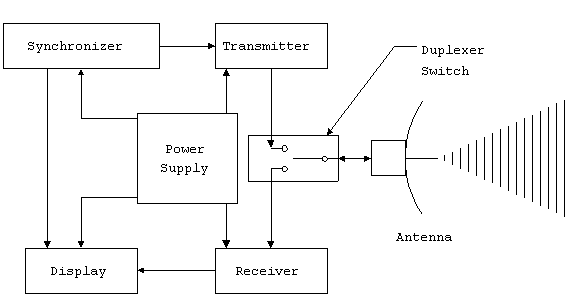Figure 3

Transmitter. The transmitter creates the radio wave to be sent and modulates it to form the pulse train.  The transmitter must also amplify the signal to a high power level to provide adequate range.  The source of the carrier wave could be a Klystron, Traveling Wave Tube (TWT) or Magnetron.  Each has its own characteristics and limitations.

2.  Receiver.  The receiver is sensitive to the range of frequencies being transmitted and provides amplification of the returned signal. In order to provide the greatest range, the receiver must be very sensitive without introducing excessive noise.  The ability to discern a received signal from background noise depends on the signal-to-noise ratio (S/N).

The background noise is specified by an average value, called the noise-equivalent-power (NEP).  This directly equates the noise to a detected power level so that it may be compared to the return.  Using these definitions, the criterion for successful detection of a target is

Pr > (S/N) NEP,

where Pr is the power of the return signal. Since this is a significant quantity in determining radar system performance, it is given a unique designation, Smin, and is called the Minimum Signal for Detection

Smin = (S/N) NEP

Since Smin, expressed in Watts, is usually a small number, it has proven useful to define the decibel equivalent, MDS, which stands for Minimum Discernible Signal.

MDS = 10 Log (Smin/1 mW)

When using decibels, the quantity inside the brackets of the logarithm must be a number without units.  I the definition of MDS, this number is the fraction Smin /1 mW.  As a reminder, we use the special notation dBm for the units of MDS,  where the "m" stands for 1 mW.  This is shorthand for decibels referenced to 1 mW, which is sometimes written as dB//1mW.

In the receiver, S/N sets a threshold for detection which determines what will be displayed and what will not.  In theory, if S/N = 1, then only returns with power equal to or greater than the background noise will be displayed.  However, the noise is a statistical process and varies randomly.  The NEP is just the average value of the noise.  There will be times when the noise exceeds the threshold that is set by the receiver.  Since this will be displayed and appear to be a legitimate target, it is called a false alarm.  If the SNR is set too high, then there will be few false alarms, but some actual targets may not be displayed known as a miss).  If SNR is set too low, then there will be many false alarms, or a high false alarm rate (FAR).

Some receivers monitor the background and constantly adjust the SNR to maintain a constant false alarm rate, and therefore all called CFAR receivers.

1.)  Pulse Integration.  The receiver takes an average return strength over many pulses.  Random events like noise will not occur in every pulse and therefore, when averaged,  will have a reduced effect as compared to actual targets that will be in every pulse.

2.)  Sensitivity Time Control (STC).  This feature reduces the impact of returns from sea state.  It reduces the minimum SNR of the receiver for a short duration immediately after each pulse is transmitted. The effect of adjusting the STC is to reduce the clutter on the display in the region directly around the transmitter.  The greater the value of STC, the greater the range from the transmitter in which clutter will be removed.  However, an excessive STC will blank out potential returns close to the transmitter.

3.) Fast Time Constant (FTC).  This feature is designed to reduce the effect of long duration returns that come from rain.  This processing requires that strength of the return signal must change quickly over it duration.  Since rain occurs over and extended area, it will produce a long, steady return.  The FTC processing will
filter these returns out of the display.  Only pulses that rise and fall quickly will be displayed.  In technical terms, FTC is a differentiator, meaning it determines the rate of change in the signal, which it then uses to discriminate pulses which are not changing rapidly.

3.  Power Supply.  The power supply provides the electrical power for all the components.  The largest consumer of power is the transmitter which may require several kW of average power.  The actually power transmitted in the pulse may be much greater than 1 kW.  The power supply only needs to be able to provide the average amount of power consumed, not the high power level during the actual
pulse transmission.  Energy can be stored, in a capacitor bank for instance, during the rest time.  The stored energy then can be put into the pulse when transmitted, increasing the peak power.  The peak power and the average power are related by the quantity called duty cycle, DC.  Duty cycle is the fraction of each transmission cycle that the radar is actually transmitting.  Referring to the pulse train in Figure 2, the duty cycle can be seen to be:

DC = PW / PRF

Synchronizer. The synchronizer coordinates the timing for range determination.

It regulates that rate at which pulses are sent (i.e. sets PRF) and resets the timing
clock for range determination for each pulse.  Signals from the synchronizer are sent

simultaneously to the transmitter, which sends a new pulse, and to the display,
which resets the return sweep.

Duplexer. This is a switch which alternately connects the transmitter or receiver to the antenna. Its purpose is to protect the receiver from the high power output of the transmitter. During the transmission of an outgoing pulse, the duplexer will be aligned to the transmitter for the duration of the pulse, PW. After the pulse has been sent, the duplexer will align the antenna to the receiver. When the next pulse is sent, the duplexer will shift back to the transmitter. A duplexer is not required if the transmitted power is low.

Antenna. The antenna takes the radar pulse from the transmitter and puts it into the air. Furthermore, the antenna must focus the energy into a well-defined beam which increases the power and permits a determination of the direction of the target. The antenna must keep track of its own orientation which can be accomplished by a synchro-transmitter. There are also antenna systems which do not physically move but are steered electronically (in these cases, the orientation of the radar beam is already known a priori).

The beam-width of an antenna is a measure of the angular extent of the
most powerful portion of the radiated energy.  For our purposes the main portion,
called the main lobe, will be all angles from the perpendicular where the power
is not less than ½ of the peak power, or, in decibels, -3 dB.  The beam-width is
the range of angles in the main lobe, so defined.  Usually this is resolved into
a plane of interest, such as the horizontal or vertical plane.  The antenna will
have a separate horizontal and vertical beam-width.  For a radar antenna, the
beam-width can be predicted from the dimension of the antenna in the plane of
interest by

q = l/L

where:
q is the beam-width in radians,
l is the wavelength of the radar, and
L is the dimension of the antenna, in the direction of interest (i.e. width or height).

In the discussion of communications antennas, it was stated that the
beam-width for an antenna could be found using q = 2l/L.  So it appears that radar
antennas have one-half of the beam-width as communications antennas. The difference
is that radar antennas are used both to transmit and receive the signal. The
interference effects from each direction combine, which has the effect of reducing
the beam-width.  Therefore when describing two-way systems (like radar) it is
appropriate to reduce the beam-width by a factor of ½ in the beam-width
approximation formula.

The directional gain of an antenna is a measure of how well the beam is
focused in all angles.  If we were restricted to a single plane, the directional
gain would merely be the ratio 2p/q.  Since the same power is distributed over a
smaller range of angles, directional gain represents the amount by which the power
in the beam is increased.  In both angles, then directional gain would be given by:

Gdir = 4p/q f

since there are 4p steradians corresponding to all directions (solid angle, measured
in steradians, is defined to be the area of the beam front divided by the range
squared, therefore a non-directional beam would cover an area of 4pR2 at distance R,

Here we used:

Sometimes directional gain is measured in decibels, namely 10 log (Gdir).
As an example, an antenna with a horizontal beam-width of 1.50 (0.025 radians) and
vertical beam-width of 20o (0.33 radians) will have:

directional gain(dB) = 10 log (4
p/ 0.025 0.333) = 30.9 dB

Example:  find the horizontal and vertical beam-width of the AN/SPS-49 long
range radar system, and the directional gain in dB. The antenna is 7.3 m wide
by 4.3 m tall, and operates at 900 MHz.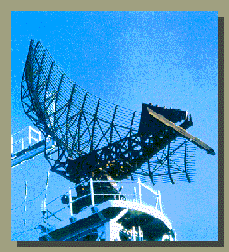The wavelength, l=c/f = 0.33 m.

Given that L= 7.3 m, then
q = l/L = 0.33/7.3 = 0.045 radians, or
q = 30.

The antenna is 4.3 m tall, so a similar calculation gives
f = 40.

The directional gain,
Gdir = 4p/(0.045  0.076) = 3638.

Expressed in decibels,
directional gain = 10 Log(3638)
= 35.6 dB.

Display. The display unit may take a variety of forms but in general is designed to present the received information to an operator. The most basic display type is called an A-scan (amplitude vs. Time delay). The vertical axis is the strength of the return and the horizontal axis is the time delay, or range. The A-scan provides no information about the direction of the target.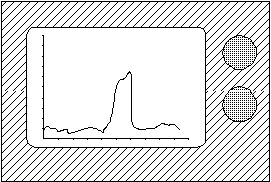Figure 4

The most common display is the PPI (plan position indicator). The A-scan information is converted into brightness and then displayed in the same relative direction as the antenna orientation. The result is a top-down view of the situation where range is the distance from the origin. The PPI is perhaps the most natural display for the operator and therefore the most widely used. In both cases, the synchronizer resets the trace for each pulse so that the range

information will begin at the origin.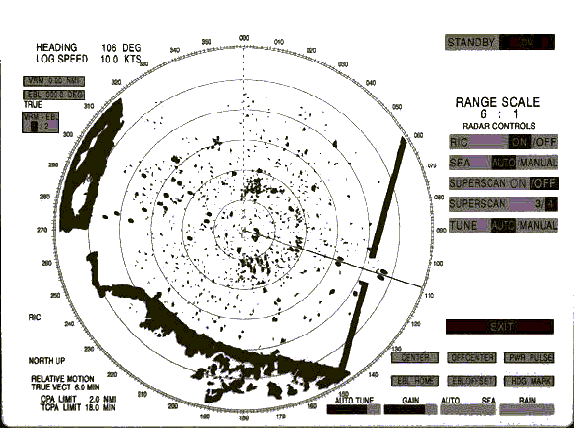Figure 5

In this example, the use of increased STC to suppress the sea clutter would be helpful.

All of the parameters of the basic pulsed radar system will affect the
performance in some way.  Here we find specific examples and quantify this dependence
where possible.

Pulse Width

The duration of the pulse and the length of the target along the radial direction
determine the duration of the returned pulse.  In most cases the length of the
return is usually very similar to the transmitted pulse. In the display unit, the
pulse (in time) will be converted into a pulse in distance.  The range of values
from the leading edge to the trailing edge will create some uncertainty in the
range to the target.  Taken at face value, the ability to accurately measure range
is determined by the pulse width.

If we designate the uncertainty in measured range as the range resolution,
RRES, then it must be equal to the range equivalent of the pulse width, namely:

RRES = c PW/2

Now, you may wonder why not just take the leading edge of the pulse as the
range which can be determined with much finer accuracy?  The problem is that it is
virtually impossible to create the perfect leading edge.  In practice, the ideal
pulse will really appear like: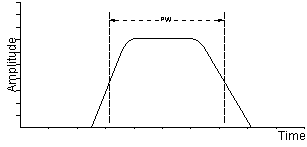Figure 6

To create a perfectly formed pulse with a vertical leading edge would require an infinite bandwidth. In fact you may equate the bandwidth, b, of the transmitter to the minimum pulse width, PW by:

PW = 1/2b

Given this insight, it is quite reasonable to say that the range can be determined no more accurately than cPW/2 or equivalently

RRES = c/4b

In fact, high resolution radar is often referred to as wide-band radar which you now see as equivalent statements. One term is referring to the time domain and the other the frequency domain. The duration of the pulse also affects the minimum range at which the radar system can detect. The outgoing pulse must physically clear the antenna before the return can be processed. Since this lasts for a time interval equal to the pulse width, PW, the minimum displayed range is then:

RMIN = c PW/2

The minimum range effect can be seen on a PPI display as a saturated or blank area
around the origin.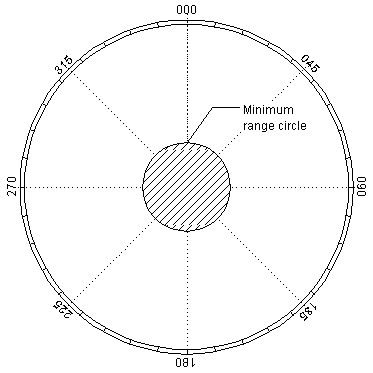Figure 7

Increasing the pulse width while maintaining the other parameters the same will also affect the duty cycle and therefore the average power. For many systems, it is desirable to keep the average power fixed. Then the PRF must be simultaneously changed with PW in order to keep the product PW x PRF the same. For example, if the pulse width is reduced by a factor of ½ in order to improve the resolution, then the PRF is usually doubled.

Pulse Repetition Frequency (PRF)

The frequency of pulse transmission affects the maximum range that can be
displayed.  Recall that the synchronizer resets the timing clock as each new pulse
is transmitted.  Returns from distant targets that do no reach the receiver until
after the next pulse has been sent will not be displayed correctly.  Since the timing
clock has been reset, they will be displayed as if the range where less than actual.
If this were possible, then the range information would be considered ambiguous.
An operator would not know whether the range were the actual range or some greater
value.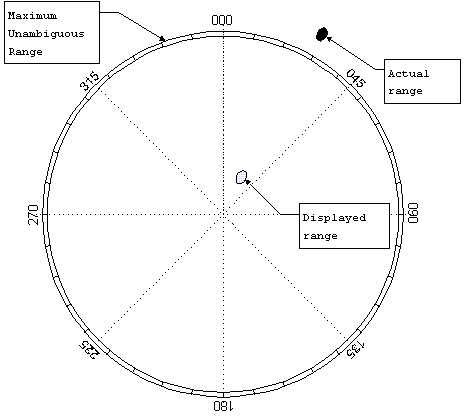Figure 8

The maximum actual range that can be detected and displayed without ambiguity, or the maximum unambiguous range, is just the range corresponding to a time interval equal to the pulse repetition time, PRT. Therefore, the maximum unambiguous range,

RUNAMB = c PRT/2 = c/(2PRF)

When a radar is scanning, it is necessary to control the scan rate so that a
sufficient number of pulses will be transmitted in any particular direction in order
to guarantee reliable detection.  If too few pulses are used, then it will more
difficult to distinguish false targets from actual ones.  False targets may be present
in one or two pulses but certainly not in ten or twenty in a row.  Therefore to
maintain a low false detection rate, the number of pulses transmitted in each
direction should be kept high, usually above ten.

For systems with high pulse repetition rates (frequencies), the radar beam
can be repositioned more rapidly and therefore scan more quickly.  Conversely, if the
PRF is lowered the scan rate needs to be reduced.  For simple scans it is easy to
quantify the number of pulses that will be returned from any particular target.  Let
t represent the dwell time, which is the duration that the target remains in the
radar's beam during each scan.  The number of pulses, N,  that the target will be
exposed to during the dwell time is:

N = t PRF

We may rearrange this equation to make a requirement on the dwell time for a particular scan

tmin = Nmin /PRF

So it is easy to see that high pulse repetition rates require smaller dwell times. For a continuous circular scan, for example, the dwell time is related to the rotation rate and the beam-width.

t = q/W

where q = beam-width [degrees] W = rotation rate [degrees/sec] which will give the dwell time in seconds. These relationships can be combined, giving the following equation from which the maximum scan rate may be determined for a minimum number of pulses per scan:

WMAX = q PRF/N

Finally, the frequency of the radio carrier wave will also have some
affect on how the radar beam propagates.  At the low frequency extremes, radar beams
will refract in the atmosphere and can be caught in "ducts" which result in long
ranges.  At the high extreme, the radar beam will behave much like visible light and
travel in very straight lines.   Very high frequency radar beams will suffer high
losses and are not suitable for long range systems.

The frequency will also affect the beam-width.  For the same antenna size,
a low frequency radar will have a larger beam-width than a high frequency one.
In order to keep the beam-width constant, a low frequency radar will need a large
antenna.

Theoretical Maximum Range Equation

A radar receiver can detect a target if the return is of sufficient strength.
Let us designate the minimum return signal that can be detected as Smin, which should
have units of Watts, W.  The size and ability of a target to reflect radar energy can
be summarized into a single term, s, known as the radar cross-section, which has units
of m2.  If absolutely all of the incident radar energy on the target were reflected
equally in all directions, then the radar cross section would be equal to the target's
cross-sectional area as seen by the transmitter.  In practice, some energy is absorbed
and the reflected energy is not distributed equally in all directions.  Therefore, the
radar cross-section is quite difficult to estimate and is normally determined by
measurement.

Given these new quantities we can construct a simple model for the radar power

Pr = Pt G 1/4pR2 s 1/4pR2 Ae

The terms in this equation have been grouped to illustrate the sequence from transmission to collection. Here is the sequence in detail:

G = r Gdir

The transmitter puts out peak power Pt into the antenna, which focuses it into a beam with gain G. The power gain is similar to the directional gain, Gdir, except that it must also include losses from the transmitter to the antenna. These losses are summarized by the single term for efficiency, r. Therefore

The radar energy spreads out uniformly in all directions. The power per unit area must therefore decrease as the area increases. Since the energy is spread out over the surface of a sphere the factor of 1/4pR2 accounts for the reduction.

The radar energy is collected by the surface of the target and reflected. The radar cross section s accounts for both of these processes.

The reflected energy spreads out just like the transmitted energy.

The receiving antenna collects the energy proportional to its effective area, known as the antenna's aperture, Ae. This also includes losses in the reception process until the signal reaches the receiver. Hence the subscript "e" for "effective." The effective aperture is related to the physical aperture, A, by the same efficiency term used in power gain, given the symbol r. So that

Ae = r A

Our criterion for detection is simply that the received power, Pr must
exceed the minimum, Smin.  Since the received power decreases with range, the maximum
detection range will occur when the received power is equal to the minimum , i.e.
Pr = Smin.  If you solve for the range, you get an equation for the maximum theoretical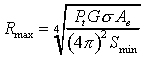Perhaps the most important feature of this equation is the fourth-root dependence. The practical implication of this is that one must greatly increase the output power to get a modest increase in performance. For example, in order to double the range, the transmitted power would have to be increased 16-fold. You should also note that the minimum power level for detection, Smin, depends on the noise level. In practice, this quantity constantly be varied in order to achieve the perfect balance between high sensitivity which is susceptible to noise and low sensitivity which may limit the radar's ability to detect targets. Example: Find the maximum range of the AN/SPS-49 radar, given the following data

Antenna Size = 7.3 m wide by 4.3 m tall
Efficiency = 80 %
Peak power = 360 kW
Cross section = 1 m2
Smin = 1  10-12 W

We know from the previous example, that the directional antenna gain,

Gdir  = 4p/qf = 4p/(.05 x .07) = 3430

The power gain,
G =  r Gdir

G = 2744.

Likewise, the effective aperture,
Ae = r = .8(7.3 x 4.3)

Ae = 25.1 m2.

Therefore the range is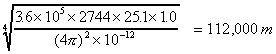, or

R = 112 km.